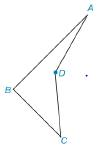Chapter 2.5, Problem 40E### Elementary Geometry for College St...

6th Edition
Daniel C. Alexander + 1 other
ISBN: 9781285195698

#### Solutions

Chapter
Section### Elementary Geometry for College St...

6th Edition
Daniel C. Alexander + 1 other
ISBN: 9781285195698
Textbook Problem
1 views

# For the concave quadrilateral ABCD, explain why the sum of the measures of the interior angles is 360 ° . (HINT: Draw B D ¯ .)To determine

To explain:

The reason that the Sum of the measure of the interior angles is 360°.

Explanation

Given:

The following figure shows the given diagram.

Figure (1)

Approach:

Draw an auxiliary segment BD¯ and mentioned the angles as shown below.

Figure (2)

Calculation:

According to the angle sum property of triangle the sum of three angles in a triangle will be 180°.

In ΔBCD,

mC+m3+m4=180°

mA+m1+m2=180°

The equation for sum of interior angles of the quadrilateral ABCD is,

S=mC+m<

### Still sussing out bartleby?

Check out a sample textbook solution.

See a sample solution

#### The Solution to Your Study Problems

Bartleby provides explanations to thousands of textbook problems written by our experts, many with advanced degrees!

Get Started

#### Find more solutions based on key concepts